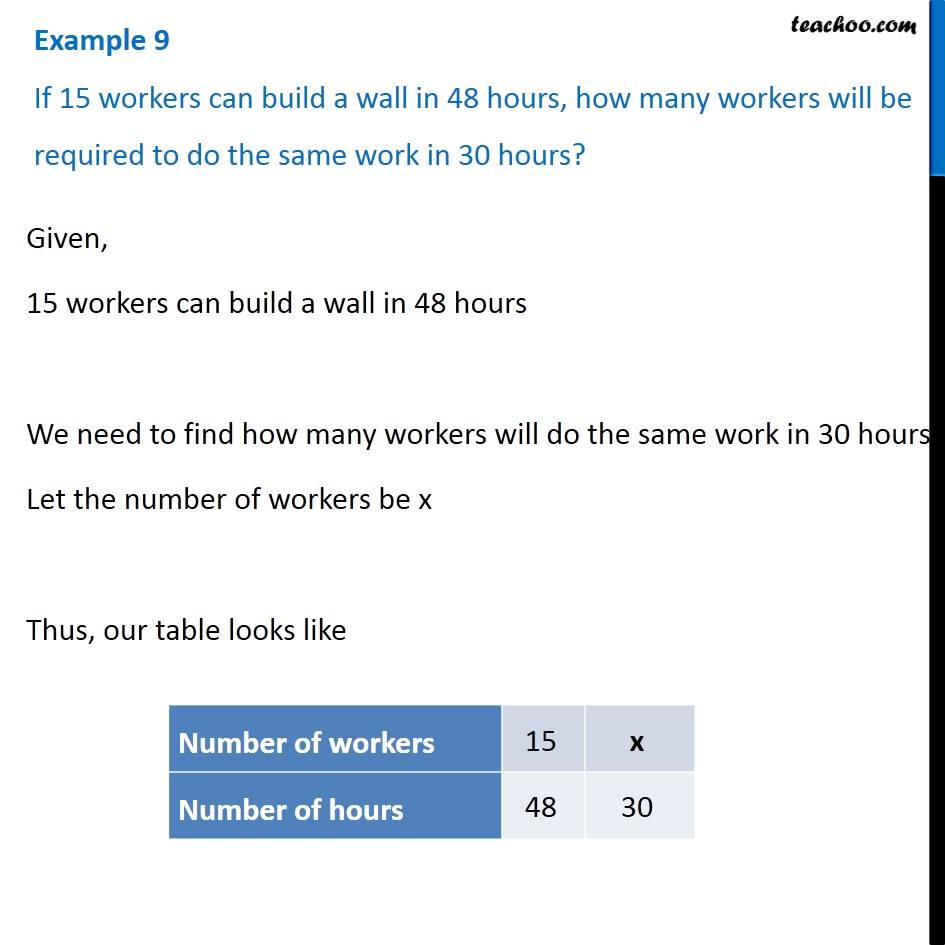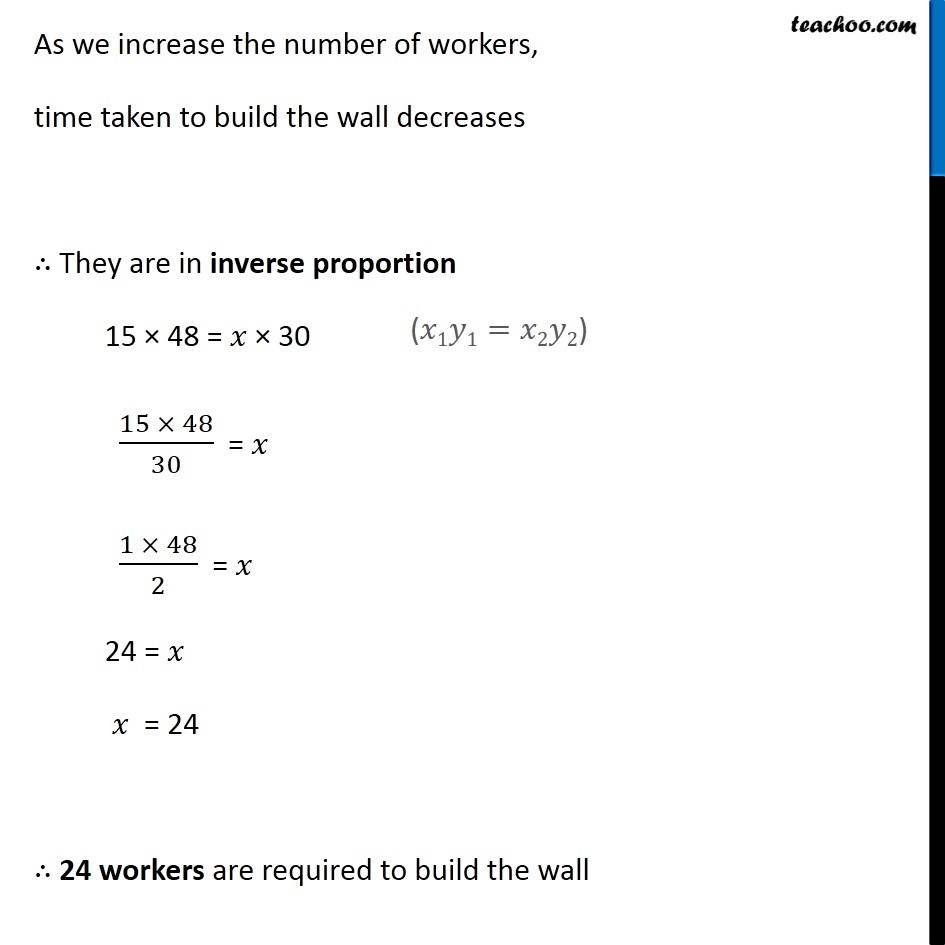Inverse Proportion

Chapter 13 Class 8 Direct and Inverse Proportions
Concept wise### Transcript

Example 9 If 15 workers can build a wall in 48 hours, how many workers will be required to do the same work in 30 hours? Given, 15 workers can build a wall in 48 hours We need to find how many workers will do the same work in 30 hours Let the number of workers be x Thus, our table looks like As we increase the number of workers, time taken to build the wall decreases ∴ They are in inverse proportion 15 × 48 = 𝑥 × 30 (15 × 48)/30 = 𝑥 (1 × 48)/2 = 𝑥 24 = 𝑥 𝑥 = 24 ∴ 24 workers are required to build the wall (𝑥1𝑦1=𝑥2𝑦2)